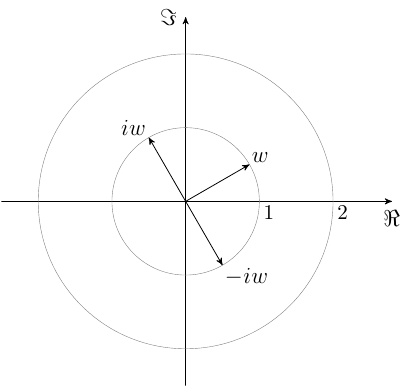# complex no.

#### jacks

##### Well-known member
If $$z$$ and $$\omega$$ are two complex no. such that $$\mid z \mid =\mid \omega \mid = 1$$ and $$\mid z+i\omega \mid = \mid z-i\omega \mid = 2$$.Then find value of $$z$$

#### Sudharaka

##### Well-known member
MHB Math Helper
If $$z$$ and $$\omega$$ are two complex no. such that $$\mid z \mid =\mid \omega \mid = 1$$ and $$\mid z+i\omega \mid = \mid z-i\omega \mid = 2$$.Then find value of $$z$$
Hi jacks,

Take, $$z=x_1+iy_1\mbox{ and }w=x_2+iy_2$$

Since, $$\displaystyle \mid z+i\omega \mid = \mid z-i\omega \mid$$,

$\mid(x_1+iy_1)+i(x_2+iy_2)\mid=\mid(x_1+iy_1)-i(x_2+iy_2)\mid$

$\Rightarrow\mid(x_1-y_2)+i(x_2+y_1)\mid=\mid(x_1+y_2)+i(y_1-x_2)\mid$

$\Rightarrow (x_1-y_2)^2+(x_2+y_1)^2=(x_1+y_2)^2+(y_1-x_2)^2$

$\Rightarrow x_{1}^{2}+y_{1}^{2}+x_{2}^{2}+y_{2}^{2}-2x_{1}y_2+2x_{2}y_1=x_{1}^{2}+y_{1}^{2}+x_{2}^{2}+y_{2}^{2}+2x_{1}y_2-2x_{2}y_1$

$\Rightarrow -2x_{1}y_2+2x_{2}y_1=2x_{1}y_2-2x_{2}y_1$

$\Rightarrow x_{1}y_2-x_{2}y_1=0$

Since, $$\mid z-i\omega \mid = 2$$,

\begin{equation}x_{1}^{2}+y_{1}^{2}+x_{2}^{2}+y_{2}^{2}+2x_{1}y_2-2x_{2}y_1=4\end{equation}

Also, $$\mid z \mid =\mid \omega \mid = 1\Rightarrow x_{1}^{2}+y_{1}^{2}+x_{2}^{2}+y_{2}^{2}=2$$

$\therefore x_{1}y_2-x_{2}y_1=1$

This is a contradiction since we have obtained $$x_{1}y_2-x_{2}y_1=0$$. There are no complex numbers $$z$$ and $$w$$ satisfying the given conditions.

•jacks

#### Evgeny.Makarov

##### Well-known member
MHB Math Scholar
Another way to see this.Since |z| = 1, the only way for |z + iw| to be 2 is for z to coincide with iw, but then |z - iw| = 0.

•jacks and Sudharaka